# Physics - Classical Mechanics - Angular motion basics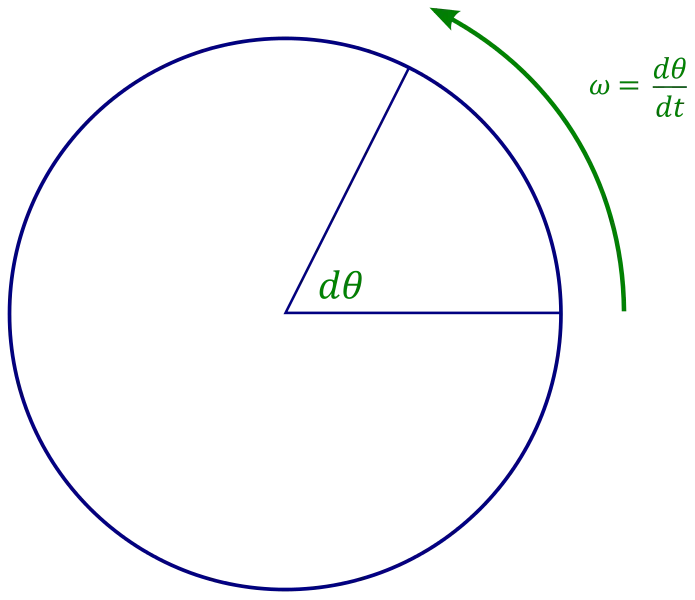[Image 1]

## Introduction

Hey it's a me again @drifter1! Today we continue with Physics and more specifically the branch "Classical Mechanics" to get into Angular motion, giving the basics of how we define position, velocity and acceleration in such motions. I guess that it's worth mentioning that some topics where already covered in circular motion, but we will get into them a little bit more in-depth now. So, without further ado, let's get straight into it!

## Angular position

Thinking about the dimensions that motion takes place, we can talk about:
• straight-line or linear motion, where a particle moves along one axis
• plane motion, where a particle moves along two axes (e.g. xy-plane)
Angular motion is a more specific case of a plane motion, where we consider the particle to be moving in a circle that is parallel to the xy-plane, staying perpendicular to the z-axis (third dimension). Therefore, we consider that there's one diameter of the circle along the x-axis and another diameter along the y-axis. Of course these diameters are perpendicular to each other.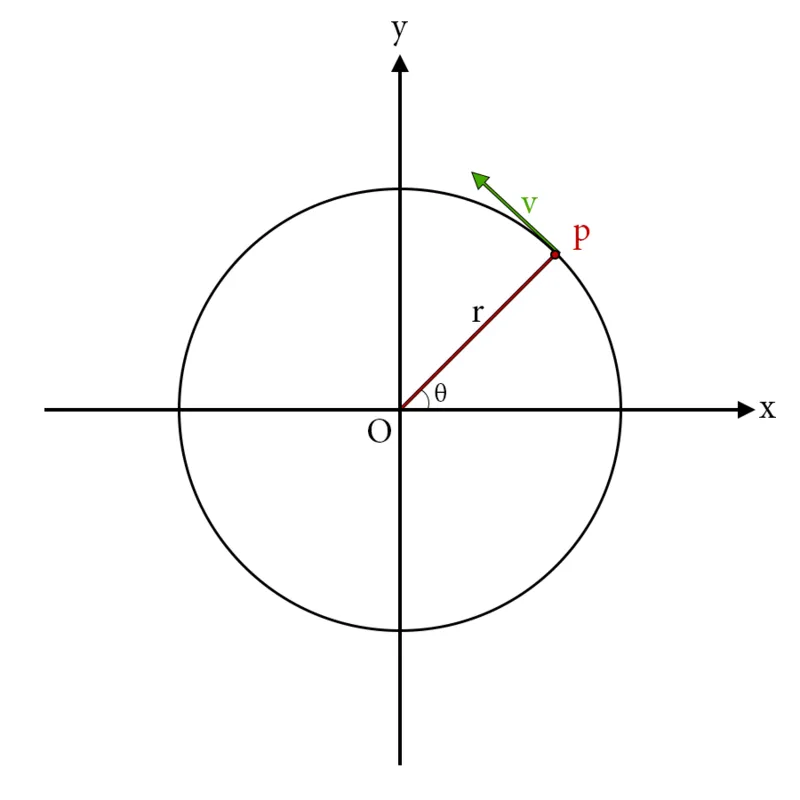[Based on Figure 1 from physicskey.com]

Let's consider the upper Figure, where a particle moves along the circle with a velocity v and being at the point p(x, y). The position of the particle can also be described by the angular angle θ that is measured from above the positive x-axis. So, clockwise rotation along the circle is considered to be negative and anti-clockwise to be positive. To know the direction we just have to find the angle difference Δθ.

The angle difference can also be calculated knowing the arc length Δs of the motion (part of the circumference a particle travelled) and radius r of the circle: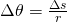So, in the end the position of the particle is determined by the angular angle θ.

## Angular velocity

In angular motions, velocity is defined as the angular displacement over time. So, if θ1 is the angular displacement/position at some time t1 and θ2 the angular displacement at time t2, the average angular velocity is: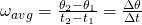As you can see, the angular velocity is denoted by the Greek letter "ω" (omega). It's calculated in rad/sec, based on the angle unit of Radians (2π rad = circle) and the time unit of Seconds. Thinking about instantaneous angular velocity, we have to let the time interval Δt approach zero. This gives us:Angular velocity is determined by the right hand rule, where you have to curl the fingers of your right hand in the direction of rotation, leaving the thumb straight along the z-axis or perpendicular along the plane of rotation (xy-plane in our case). The thumb gives us the direction. If the particle moves clockwise, the angular velocity "goes upward". In the case of anti-clockwise motion, the angular velocity will "go into the page".
Doing some calculations using this equation, we can end up with the following equation that gives us the tangential speed of the particle, using the radius and angular velocity: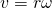Let's also write the vector-form of this equation: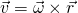This equation, shows us why the angular velocity is in the third dimension...

## Angular acceleration

As always acceleration defines the change in speed over time. So, in this case, angular acceleration is defined as the change in angular velocity over time:To find the direction of angular acceleration, you can't use the right hand thumb rule of before. Here you have to find the difference of the angular velocities. So, if the particle's linear speed increases (in clockwise or anti-clockwise direction) then the angular velocity and acceleration lie in the same direction. If the linear speed decreases then the angular velocity and acceleration lie in opposite directions.

The centripetal (towards the centre) and tangential acceleration of the particle can be calculated using the following equations, that can be proven using the previous equations of velocity:## RESOURCES:

### References

1. https://www.physicskey.com/20/angular-position-velocity-and-acceleration
2. https://courses.lumenlearning.com/boundless-physics/chapter/velocity-acceleration-and-force/
3. https://phys.libretexts.org/Bookshelves/University_Physics/Book%3A_University_Physics_(OpenStax)/Map%3A_University_Physics_I_-_Mechanics%2C_Sound%2C_Oscillations%2C_and_Waves_(OpenStax)/10%3A_Fixed-Axis_Rotation__Introduction/10.1%3A_Rotational_Variables

### Images

1.2. https://www.physicskey.com/20/angular-position-velocity-and-acceleration

## Final words | Next up

This is actually it for today's post! Next time we will start getting into the rotation of objects, starting off with angular velocity and acceleration...

See ya!Keep on drifting!

H2
H3
H4
3 columns
2 columns
1 column